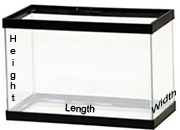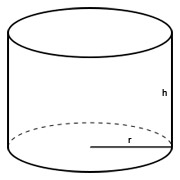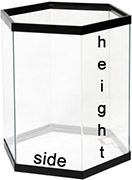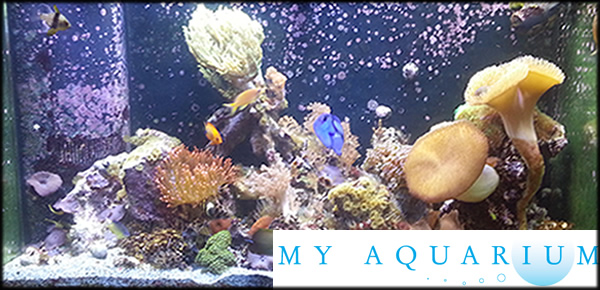Aquarium and Fish Tank Volume Calculator

This page will allow you to easily determine the volume of your aquarium using its dementions. This utility gives you the aquarium gallons using inches, but if you need to convert using centimeters or liters there are converters at the bottom that will help you do that.

The calculators below can be used to calculate the volume of water in square, rectangular, hexagonal, or cylindrical aquariums. Please note that all results in aquarium size are estimates of your fish tank volume based only on your empty fish tank volume. These estimates do not take into account any decorations, gravel, sand, rock, or other objects that may be inside the aquarium. make a minor allowance for such things.

Aquarium Size And Unit Converter Calculators

*The aquarium calculators here use javascript. They wont work if javascript isn't enabled in your internet browser.

*Numbers round up to two decimals places upon conversion.

Calculate A Square or Rectangle Fish Tank VolumeFish Tank Length in Inches:
Fish Tank Width in Inches:
Fish Tank Height in Inches:
Aquarium Size is: US Gallons
Aquarium Size is: UK Gallons

Calculate Cylinder Aquarium VolumeFish Tank Height (h) in Inches:
Fish Tank Radius (r) in Inches:
Aquarium Size is: US Gallons
Aquarium Size is: UK Gallons
Aquarium Size is: Liters

Calculate Hexagon Aquarium VolumeFish Tank Height in Inches:
Length of One Side in Inches:
Aquarium Size is: US Gallons
Aquarium Size is: UK Gallons
Aquarium Size is: Liters

Enter °F: = °C

Enter °C: = °F

Convert Gallons to Liters

Enter Gallons: = Liters

Convert Liters to Gallons

Enter Liters: = Gallons

Convert Inches to Centimeters

Enter Inches: = Centimeters

Convert Centimeters to Inches

Enter Centimeters: = Inches

Convert UK Gallons to US Gallons

Enter UK Gallons: = US Gallons

Convert US Gallons to UK Gallons

Enter US Gallons: = UK Gallons